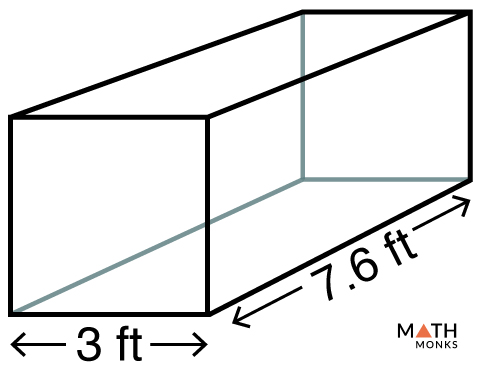# Square Prism

A square prism is a three-dimensional solid consisting of two identical square bases joined together by four rectangular faces.  It has 6 faces (2 square and 4 rectangular), 12 edges, and 8 vertices.

Toothpaste packs, eye drops or liquid medicine packs, and bar magnets are some common examples of square prisms we see in our daily life.

A 2-dimensional net can be used to construct a square prism to understand its shape. Generally a net is made from paper and cut in a manner such that it can be folded and modified into 3-D shape.

A square prism can be right or oblique depending on the alignment of its bases.

## Right Square Prism

A right square prism is a prism in which the lateral faces are perpendicular to its bases. Thus, the lateral faces are rectangular. Therefore, the bases are aligned perfectly above one another when the prism rests on its base.

## Oblique Square Prism

An oblique square prism is a slanted prism in which the lateral faces are not perpendicular to its bases. Thus, the lateral faces are parallelogram-shaped. Therefore, the bases do not appear above one another when the prism rests on its base.

## Formulas

### Surface Area

The formula to calculate the total surface area is given below:

Total Surface Area (TSA) = 2a2 + 4al, here a = apothem, b = base edge, l = length

Alsosince Lateral Surface Area (LSA) = Base perimeter height

Here, Base Perimeter = 5 ⨯ base edge ⨯ length = 4al

Lateral Surface Area (LSA) = 4al

Thus, we can write

Total Surface Area (TSA) = 2a2 LSA

Let us solve some examples to understand the concept better.

Find the lateral and the total surface area and volume of a square prism with a square face of base edge 6 in and length 9 in.

Solution:

As we know,
Lateral Surface Area (LSA) = 4al, here a = 6 in, l = 9 in
LSA = 4 × 6 × 9
= 216 in2
Total Surface Area (TSA) = 2a2 LSA, here a = 6 in, LSA = 216 in2
TSA = 2 × (6)2 + 216
= 288 in2Find the lateral surface area of a square prism given in the figure.

Solution:

As we know,
Lateral Surface Area (LSA) = 4al, here a = 3 ft, l = 7.6 ft
LSA = 4 × 3 × 7.6
= 91.2 ft2

### Volume

The volume of a square prism is its space in the three-dimensional plane. The formula is given below:

Volume (V) = a2l, here a = base edge, l = length

Let us solve an example to understand the concept better.

Find the volume of a square prism with a base edge of 7 cm and length of 11 cm.

Solution:

As we know,
Volume (V) = a2l, here a = 7 cm, l = 11 cm
V = 72 × 11
= 539 cm3

• More Resources: